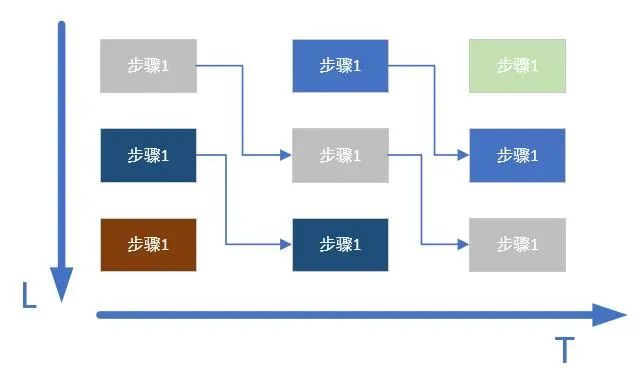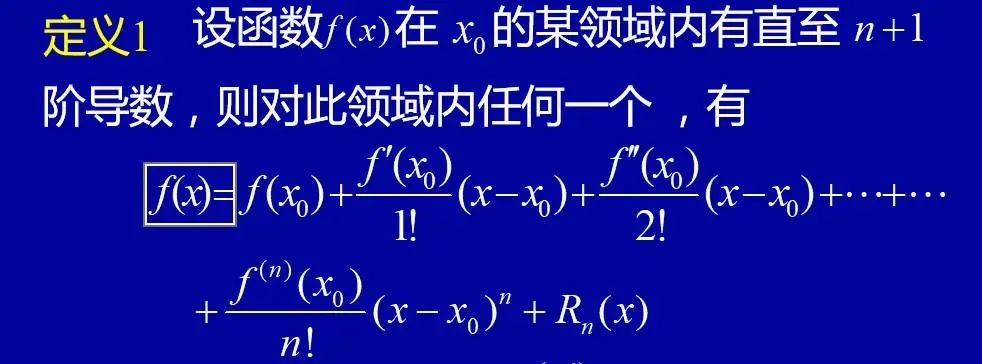# AN EMBEDDED GROUP

### 1. 算法系统结构

#### 1.1 流水线结构#### 1.2 并行阵列结构

``````
|--Data1--->Data1-->Data1

|

InputData------Data2--->Data2-->Data2

|

|--Data3--->Data3-->Data3
``````

### 2.算法转换

#### 2.1 定常数转换

``````
ex1:

dout = din * 255
``````

``````
dout = ( din << 8 ) - din

ex2:

dout = din * 11
``````

``````
dout = din * 2^2 + din * 2^3 - din * 2^0

= din * (2^2 + 2^3 - 2^0)
``````

#### 2.2 不等式等效转换

``````
ex1:

√a￣  < b, a > 0
``````

``````
b^2 > a

ex2:

a/b > c/b (b > 0, d > 0)
``````

``````
a * d > b * c
``````

#### 2.3 取近似值

``````
assign dout = din?(dout[-1~4] + 1) : (dout[-1~4])
````````````
1/3 = 1/4 * (1 + 1/4 + 1/16 + 1/64 + 1/256)
``````